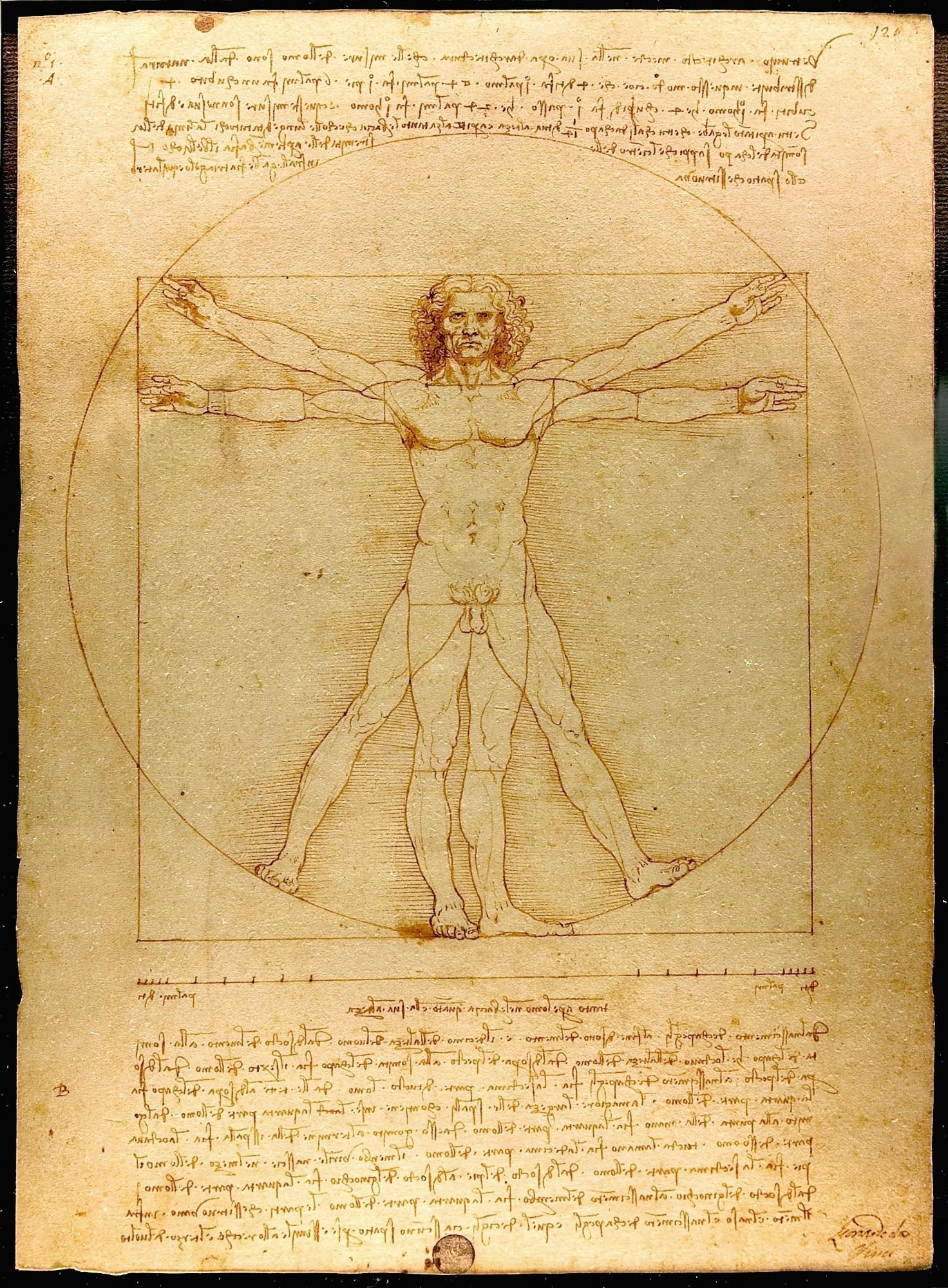# The Logarithmic Ape Index

The Ape Index (aka Gorilla Index) is a well-known term in the climbing community, and one that is often quoted, albeit primarily by compulsive, male, beta-sprayers trying to impress in compensation for not knowing how to climb.

While it is often speculated that higher Ape Indices correlate with better climbing performance, the actual merits of the figure are highly questionable. Nonetheless, it has become such a household term that it’s likely here to stay.Vitruvian Man by Leonardo da Vinci (circa 1490) popularised the notion of the ‘ideal man’ fitting perfectly into a square, with matching height and wingspan.

There are multiple, inconsistent definitions for the Ape Index, one based on the difference between height and wingspan, the other based on their ratio.

These inconsistent definitions complicate objective interpretation. Others, on the other hand, like Billy Jackson here, are unaware of the distinction between division and subtraction altogether, which brings into question whether I should be attending their firearm safety course.

If we are to continue referring to the Ape Index, it is important to employ a standardised formalism with consistent interpretation.

There are three desirable mathematical properties we would like an intuitive and consistent measure for the Ape Index to exhibit:

• Scale-invariance: the measure is preserved across individuals exhibiting the same physical ratios but of different physical sizes, such that someone preserves their index if their body ratios are preserved as they grow.
• Unitless: the measure is independent of the choice of measurement units, thereby mitigating culture wars between the United States and everyone else because the Americans are offended at being perceived less apey for refusing to use the metric system.
• Anti-symmetric: negating the measure’s value corresponds to interchanging height and wingspan, equivalently rotating the frame by 90°, i.e $A(w,h)=-A(h,w)$. Hence, the Vitruvian Man fitting a perfect square must identically have value 0.

The two alternate definitions of the Ape Index previously employed exhibit some of these characteristics, but neither exhibits all.

The first method for defining the Ape Index is as the wingspan-to-height ratio,

$A_1(w,h) = \frac{w}{h}.$

This definition exhibits scale-invariance and is unitless, however it suffers the drawback that it is not anti-symmetric. Using this measure, interchanging height and wingspan implements the transformation $A_1\to A_1^{-1}$, as opposed to $A_1\to -A_1$.

The second method for defining the Ape Index is as the difference between wingspan and height,

$A_2(w,h) = w-h.$

This definition is clearly anti-symmetric, but neither scale-invariant nor unitless.

We would ideally like a measure that exhibits all three desirable properties: scale-invariance, unitless and anti-symmetric. We achieve this by introducing the Logarithmic Ape Index (LAI), defined as follows,

$A_L(w,h) = \beta\,\mathrm{log}\left(\frac{w}{h}\right) = \beta\,[\mathrm{log}(w)-\mathrm{log}(h)],$

where $\beta>0$ is a calibration parameter, and by convention we employ the natural logarithm. Upon inspection this inherits the structural properties of both previously defined measures. From the left hand side it is both scale-free and unitless, and from the right hand side anti-symmetric. This algebraic form naturally satisfies all three desired properties based on the characteristics of the logarithmic function.

The parameter $\beta$ is specified by calibrating against a chosen point of reference. We will do so by adopting the convention that an LAI of $A_L=10$ corresponds to an adult gorilla, where maximum heights and wingspans of $h=1.8\mathrm{m}$ and $w=2.6\mathrm{m}$ have been reported respectively. This yields the Gorilla coefficient,

$\beta_G = \frac{10}{\mathrm{log}(w)-\mathrm{log}(h)}= \frac{10}{\mathrm{log}(2.6)-\mathrm{log}(1.8)}\approx 27.19.$

Gorillas, however, are an unfair point of comparison for humans, given how exceedingly apey they are. We therefore also provide a second parameterisation for the LAI using Alex Honnold — arguably the world’s most successful ape — as a human baseline, similarly defined to yield the Honnold coefficient,

$\beta_H = \frac{10}{\mathrm{log}(w)-\mathrm{log}(h)}= \frac{10}{\mathrm{log}(188)-\mathrm{log}(180)}\approx 229.96,$

where $h=180\mathrm{cm}$ and $w=188\mathrm{cm}$.

You can use my free online calculator below to calculate your Logarithmic Ape Index.

*The beta coefficient is not to be confused with the beta-spray coefficient, which scales inverse proportionately to the beta coefficient, $\beta_S=1/\beta$.

## Appendix

Below we reproduce the LAIs of some notable apes using both the Gorilla and Honnold coefficients.

Gorilla Honnold
Gorilla 10 84.56
Tomoa Narasaki 1.55 13.14
Jan Hojer 1.41 11.92
Alex Honnold 1.18 10
Emily Harrington 1.02 8.62
Jongwon Chon 0.91 7.67
Chris Sharma 0.88 7.42
Alex Puccio 0.84 7.08
Stefano Ghisolfi 0.63 5.35